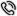服务热线4000-186-360

# RSA 算法的描述

RSA 算法公开密钥的产生
(1) 选择两个大素数p 和q;
(2) 计算n= p @ q 和z= (p- 1) @ (q- 1) ;
(3) 选择一整数e, 要满足0< e< z , 且gcd(z, e) = 1;
(4) 公开密钥PK= { e, n} 。

RSA 算法私有密钥的产生

1 Euclid 扩展算法

RSA 算法中的私有密钥通常利用Euclid 扩展算法来计算。Euclid 扩展算法在数论中应用广泛, 它被用来求解形如ax=b(mod n) 的方程。

Euclid 扩展算法首先确定两个整数x 和y, 满足:xa+ yb= 1。

(1) 初始化a1= 1, a2 = 1,b1= 0,b2 = 1;
(2) n1= q* n2 + r;
(3) If (r= = 0) gcd( n1, n2) = n2 , a= a2 , b=b2 , 算法结束;
(4) Else n1= n2, n2= r, t= a2, a2= a1- q*a2 , a1 = t,t= b2 , b2 = b1 - q* b2 ,b1 = t, 转(2) 。2 扩展算法解出RSA 算法中的私钥

RSA 中的私钥d 要满足deS 1(mod z) , d是e在模z下的乘法逆元, 因为e与z互素, 由模运算可知, 它的乘法逆元一定存在。这个方程可以利用Euclid 扩展算法解出。于是得到私钥SK={d, n} 。

3 RSA 算法中的加密和解密

4 RSA 算法实例

B 在收到密文c= 123 后, 利用只有自己知道的秘密密钥计算: m= c mod n = 123的103次方mod143= 85, 所以, B 可以得到A 发来的真正的信息m= 85, 实现了解密。